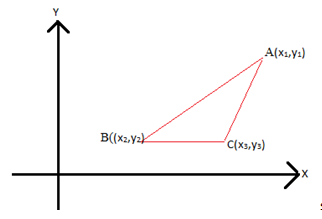Checkout JEE MAINS 2022 Question Paper Analysis : Checkout JEE MAINS 2022 Question Paper Analysis :

# Determinant To Find Area Of A Triangle

We know what a determinant is, let us know how to use Determinant to find Area of a Triangle

Suppose we are given three points in the Cartesian plane as

$$\begin{array}{l} (x_1 , y_1) , (x_2,y_2) \space and \space (x_3 , y_3) \end{array}$$
. The area of the triangle obtained by joining these points is given by,

$$\begin{array}{l} \alpha = \frac 12 [ x_1 (y_2 – y_3) + x_2 (y_3 – y_1) + x_3 (y_1 – y_2)] \end{array}$$

Where

$$\begin{array}{l} \alpha \end{array}$$
denotes the area of the triangle and
$$\begin{array}{l} (x_1 , y_1) , (x_2,y_2) \space and \space (x_3 , y_3) \end{array}$$
,  represent the vertices of the triangle.The formula for finding area could be represented in the form of determinants as given below.

$$\begin{array}{l} \alpha =\frac 12 \left| \begin{matrix} x_1 & y_1 & 1\cr x_2 & y_2 & 1 \cr x_3 & y_3 & 1 \cr \end{matrix} \right| \end{array}$$

As we know the value of a determinant can either be negative or a positive value but since we are talking about area and it can never be taken as a negative value, therefore we take the absolute value of the determinant so obtained.

If the area of the triangle is already given then we make use of both the positive and negative values of the determinant.

Also, if three points are collinear we would be left with a straight line instead of a triangle and as the area enclosed by a straight line is zero hence the value of the determinant will also be zero.

Keeping the above-mentioned points in mind,  let us try to expand the determinant which denoted the area by using determinant expansion techniques using minors and cofactors.

Therefore,

$$\begin{array}{l} \alpha = \frac 12 [ x_1 (y_2 – y_3) + x_2 (y_3 – y_1) + x_3 (y_1 – y_2)] \end{array}$$

Hence we see that how determinants are applied to make calculations easy. Now let us try our hands at this application of determinants to find out the area of triangles.

## Example To find Area of Triangle using Determinant

Example: Find out the area of the triangle whose vertices are given by A(0,0) , B (3,1) and C (2,4).

Solution: Using determinants we can easily find out the area of the triangle obtained by joining these points using the formula

$$\begin{array}{l} \alpha = \frac 12\left| \begin{matrix} x_1 & y_1 & 1\cr x_2 & y_2 & 1 \cr x_3 & y_3 & 1 \cr \end{matrix} \right| \end{array}$$
.

Substituting the respective values in the determinant we have

$$\begin{array}{l} \alpha = \frac 12\left| \begin{matrix} 0 & 0 & 1\cr 3 & 1 & 1 \cr 2 & 4 & 1 \cr \end{matrix} \right| \end{array}$$

Expanding the above determinant by using expansion techniques of determinant we get,

$$\begin{array}{l} \alpha = \frac 12 [ 0 (1-4) – 0(3-2) + 1 (12-2)] \end{array}$$

$$\begin{array}{l} \Rightarrow \alpha = 5 units \end{array}$$
<